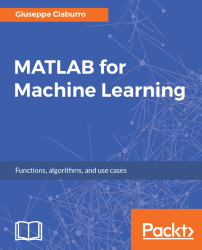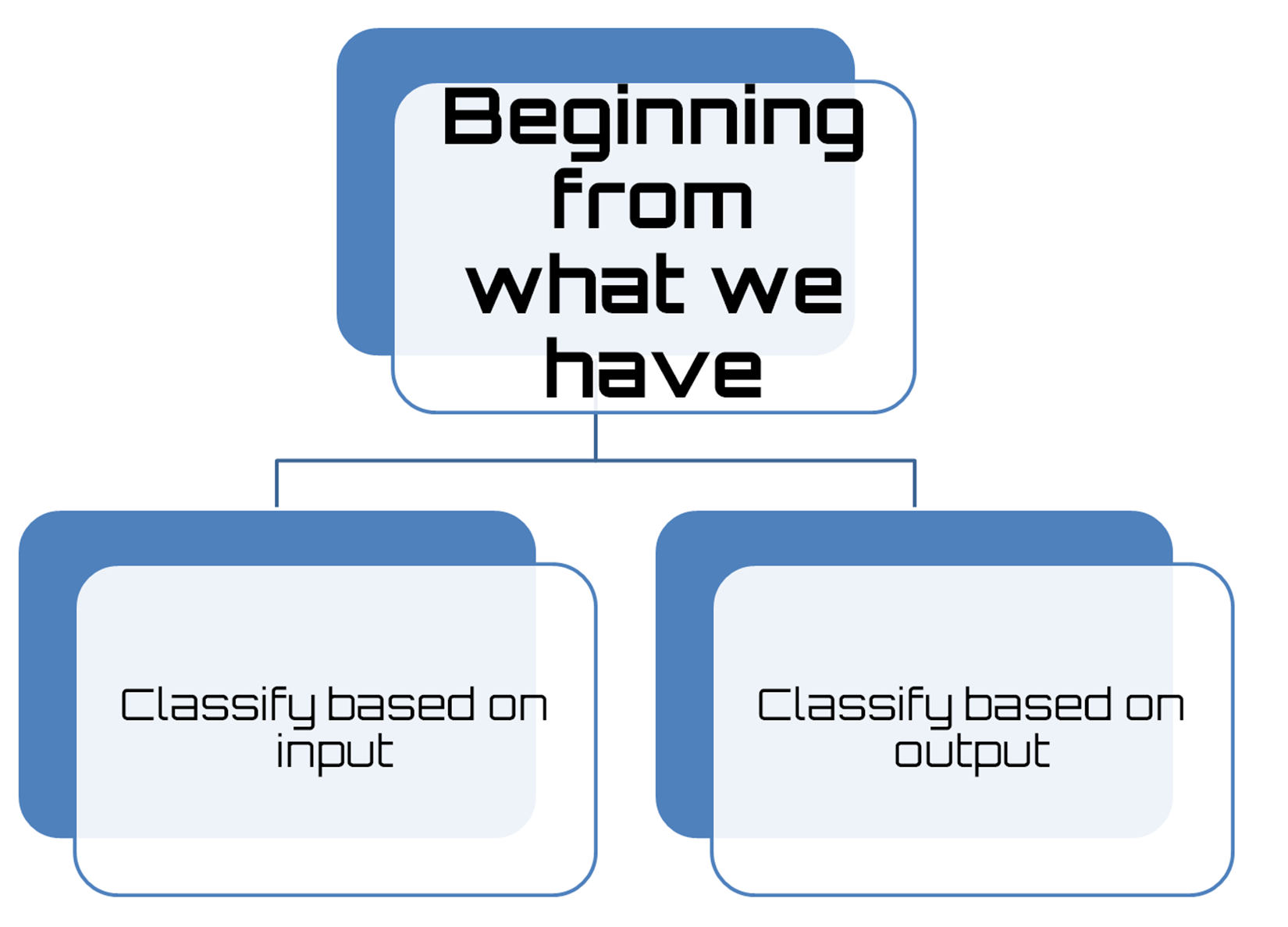•#### MATLAB for Machine Learning#### Overview of this book

MATLAB is the language of choice for many researchers and mathematics experts for machine learning. This book will help you build a foundation in machine learning using MATLAB for beginners. You’ll start by getting your system ready with t he MATLAB environment for machine learning and you’ll see how to easily interact with the Matlab workspace. We’ll then move on to data cleansing, mining and analyzing various data types in machine learning and you’ll see how to display data values on a plot. Next, you’ll get to know about the different types of regression techniques and how to apply them to your data using the MATLAB functions. You’ll understand the basic concepts of neural networks and perform data fitting, pattern recognition, and clustering analysis. Finally, you’ll explore feature selection and extraction techniques for dimensionality reduction for performance improvement. At the end of the book, you will learn to put it all together into real-world cases covering major machine learning algorithms and be comfortable in performing machine learning with MATLAB.
Title PageCreditsForewordwww.PacktPub.comCustomer FeedbackPrefaceFree Chapter
Getting Started with MATLAB Machine LearningImporting and Organizing Data in MATLABFrom Data to Knowledge DiscoveryFinding Relationships between Variables - Regression TechniquesPattern Recognition through Classification AlgorithmsIdentifying Groups of Data Using Clustering MethodsSimulation of Human Thinking - Artificial Neural NetworksImproving the Performance of the Machine Learning Model - Dimensionality ReductionMachine Learning in Practice## Choosing the right algorithm

In the previous section, we learned the difference between various types of machine learning algorithms. So, we understood the basic principles that underlie the different techniques. Now it's time to ask ourselves the following question: What is the right algorithm for my needs?Unfortunately there is no common answer for everyone, except the more generic: It depends. But what does it depend on? It mainly depends on the data available to us: the size, quality, and nature of the data. It depends on what we want to do with the answer. It depends on how the algorithm has been expressed in instructions for the computer. It depends on how much time we have. There is no best method or one-size-fits-all. The only way to be sure that the algorithm chosen is the right one is to try it.

However, to understand what is most suitable for our needs, we can perform a preliminary analysis. Beginning from what we have (data), what tools we have available (algorithms), and what objectives we set for ourselves (the results), we can obtain useful information on the road ahead.

If we start from what we have (data), it is a classification problem, and two options are available:

• Classify based on input: We have a supervised learning problem if we can label the input data. If we cannot label the input data but want to find the structure of the system, then it is unsupervised. Finally, if our goal is to optimize an objective function by interacting with the environment, it is a reinforcement learning problem.
• Classify based on output: If our model output is a number, we have to deal with a regression problem. But it is a classification problem if the output of the model is a class. Finally, we have a clustering problem if the output of the model is a set of input groups.

The following is a figure that shows two options available in the classification problem:Figure 1.9: Preliminary analysis

After classifying the problem, we can analyze the tools available to solve the specific problem. Thus, we can identify the algorithms that are applicable and focus our study on the methods to be implemented to apply these tools to our problem.

Having identified the tools, we need to evaluate their performance. To do this, we simply apply the selected algorithms on the datasets at our disposal. Subsequently, on the basis of a series of carefully selected evaluation criteria, we carry out a comparison of the performance of each algorithm.